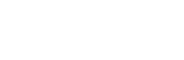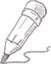#2019-03-15   阅读量: 215

## nls在一些数据子集上失败，但在其他类似的子集上没有失败

good_data = data.frame(y = c(8.46,6.87,5.81,6.62,5.85,5.79,4.83,4.94,4.95,5.27,5.05,5.38,5.08,3.98),

x = c(2,6,6,7,7,8,9,10,12,13,14,15,16,17))

bad_data = data.frame(y = c(8.99,5.86,5.32,5.74,5.41,5.04,4.66,4.52,4.18,4.66,5.38,5.46,5.21,5.37,4.89),

x = c(2,6,6,7,7,8,9,10,11,12,13,14,15,16,17))

fit = nls(y ~ SSasymp(x, Asym, R0, lrc), data = good_data)

``sp <- with(bad_data, spline(x, y))fit2sp <- nls(y ~ SSasymp(x, Asym, R0, lrc), data = sp)fit2sp``

``Nonlinear regression model  model: y ~ SSasymp(x, Asym, R0, lrc)   data: sp   Asym      R0     lrc  5.0101 22.1915 -0.2958  residual sum-of-squares: 5.365Number of iterations to convergence: 0 Achieved convergence tolerance: 1.442e-06``

``plot(y ~ x, bad_data)points(y ~ x, sp, pch = 20)fit2sp <- nls(y ~ SSasymp(x, Asym, R0, lrc), data = sp)lines(fitted(fit2sp) ~ x, sp, col = "red")``

53.7385 3 4 关注作者 收藏

## 评论(0)